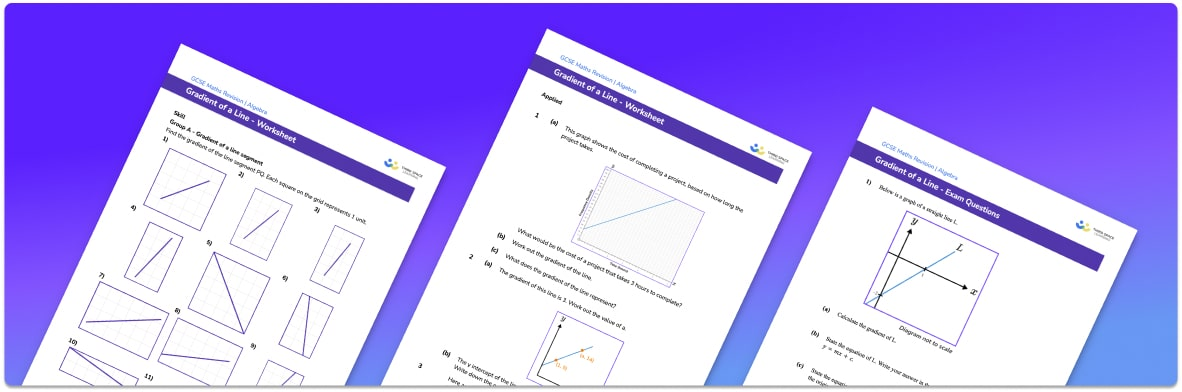# Gradient Of A Line Worksheet• Section 1 of the gradient of a line worksheet contains 20+ skills-based gradient of a line questions, in 3 groups to support differentiation
• Section 2 contains 3 applied gradient of a line questions with a mix of worded problems and deeper problem solving questions
• Section 3 contains 3 foundation and higher level GCSE gradient of a line exam questions
• Answers and a mark scheme for all gradient of a line questions are provided
• Questions follow variation theory with plenty of opportunities for students to work independently at their own level
• All questions created by fully qualified expert secondary maths teachers
• Suitable for GCSE maths revision for AQA, OCR and Edexcel exam boards

• This field is for validation purposes and should be left unchanged.

You can unsubscribe at any time (each email we send will contain an easy way to unsubscribe). To find out more about how we use your data, see our privacy policy.

### Gradient of a line at a glance

The gradient of a line tells us how steep that line is, the bigger the gradient, the steeper the line. A line with a positive gradient slopes upwards from left to right and a line with a negative gradient slopes downwards. When the equation of a straight line is written in the form y=mx+c, the value m is the gradient of the line. Straight line graphs are also known as linear graphs as their equations contain terms in x,y and constants only, with no higher powers of x or y.

The gradient of a straight line can be calculated by taking any two points on the line, subtracting the y values, subtracting the x values and then dividing them.

Since the gradient of a line tells us how steep the line is, parallel lines have equal gradients. The gradients of perpendicular lines – lines which meet at right angles – are negative reciprocals of each other.

Horizontal and vertical lines have slightly adapted equations. Lines of the form y=a (where a is a constant) are straight horizontal lines with a gradient of 0. Lines of the form x=a (where a is a constant) are straight vertical lines and have a gradient of ∞.

When drawing straight line graphs, we can either use a table of values calculated using the equation of the line, or we can use information about the y intercept and the gradient of the line. The y intercept tells us the y coordinate of the point where the line crosses the y axis and the gradient tells us how many steps to go up for every one we go across. We can start drawing our line from the given point on the y axis and move across and up/down by the appropriate amount between each point on the line.

Looking forward, students can then progress to additional straight line graphs worksheets and on to more algebra worksheets, for example a simplifying expressions worksheet or simultaneous equations worksheet.For more teaching and learning support on Algebra our GCSE maths lessons provide step by step support for all GCSE maths concepts.

## Do you have KS4 students who need more focused attention to succeed at GCSE?There will be students in your class who require individual attention to help them succeed in their maths GCSEs. In a class of 30, it’s not always easy to provide.

Help your students feel confident with exam-style questions and the strategies they’ll need to answer them correctly with our dedicated GCSE maths revision programme.

Lessons are selected to provide support where each student needs it most, and specially-trained GCSE maths tutors adapt the pitch and pace of each lesson. This ensures a personalised revision programme that raises grades and boosts confidence.

Find out more